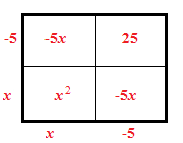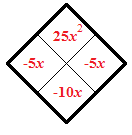### Home > CAAC > Chapter 8 > Lesson 8.3.2 > Problem8-106

8-106.

Solve the following quadratic equations by factoring and using the Zero Product Property. Then check your solutions. Homework Help ✎

1. $x^2−10x+25=0$

Use a generic rectangle and a diamond problem to help you factor the equation.$x=5$

1. $0=3x^2+17x−6$

See part (a).

1. $3x^2−2x=5$

Rewrite the equation so that it is equal to $0$.

$\textit{x}=\frac{5}{3},\ \textit{x}=-1$

1. $16x^2−9=0$

You should notice that this is a difference of squares.